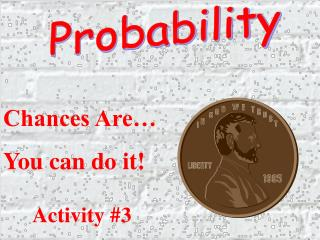DownloadDownload PresentationProbability

# Probability

Download Presentation## Probability

- - - - - - - - - - - - - - - - - - - - - - - - - - - E N D - - - - - - - - - - - - - - - - - - - - - - - - - - -
##### Presentation Transcript

1. Probability Chances Are… You can do it! Activity #3

2. DRAWING MARBLES A jar contains two red marbles, three blue marbles, and four green marbles. Niki draws one marble from the jar, and then Tom draws a marble from those remaining. What is the probability that Niki draws a green marble AND Tom draws a blue marble? Express your answer as a common fraction.

3. # of desired outcomes # of total possible outcomes REMEMBER... SO... Niki has a 4/9 chance of drawing a green and Tom has a 3/8 chance of drawing a blue.

4. # of desired outcomes # of total possible outcomes REMEMBER... SO... Niki has a 4/9 chance of drawing a green and Tom has a 3/8 chance of drawing a blue.

5. 4/9 x 3/8 = 12/72 or 1/6 There is a 1/6 chance that Niki will draw a green marble AND Tom will draw a blue marble. End of Activity So...